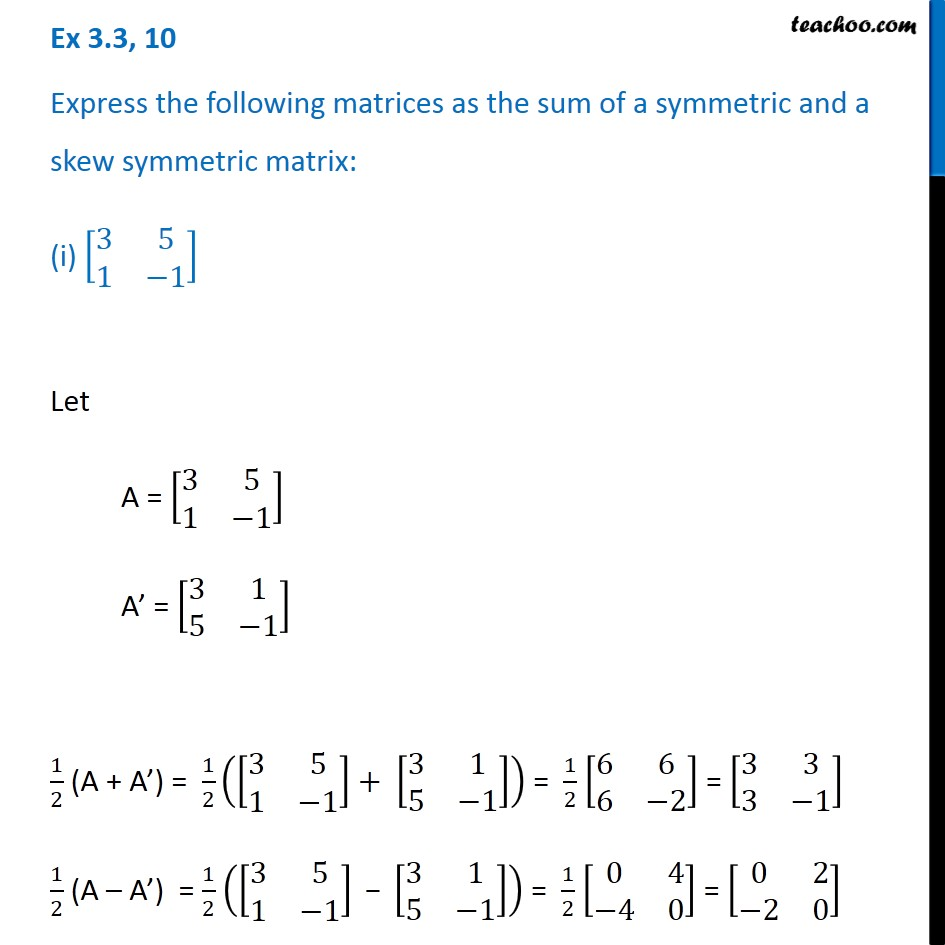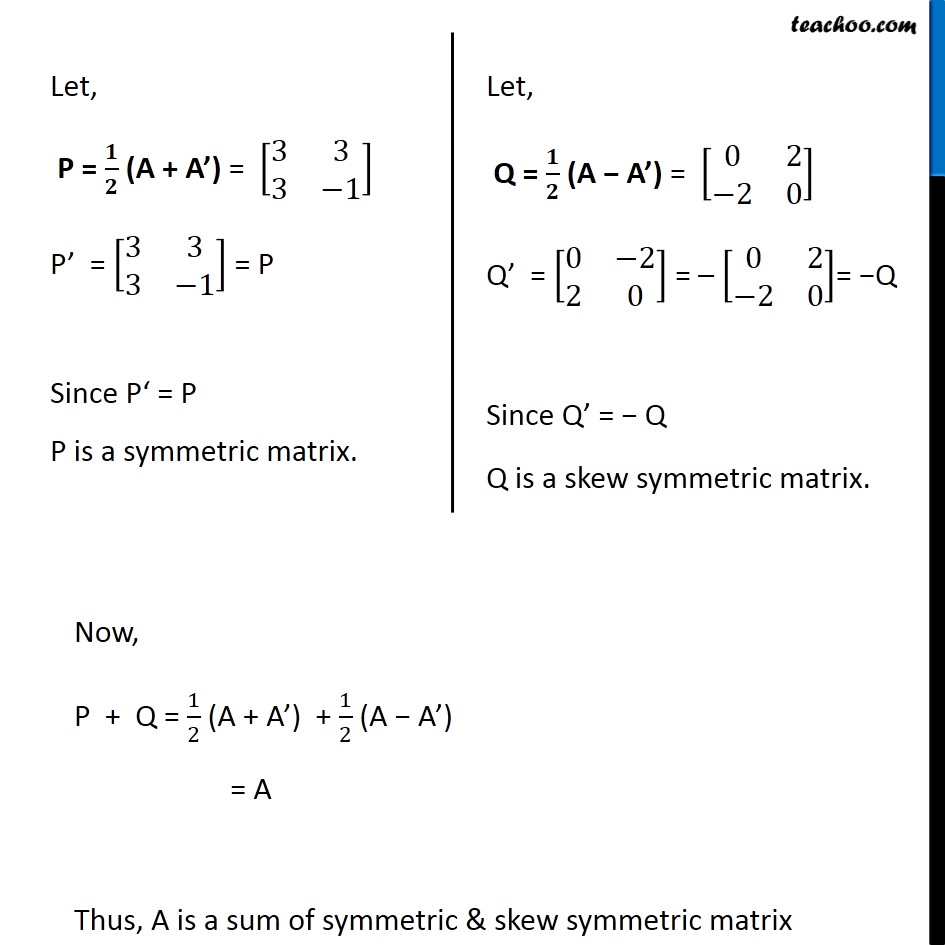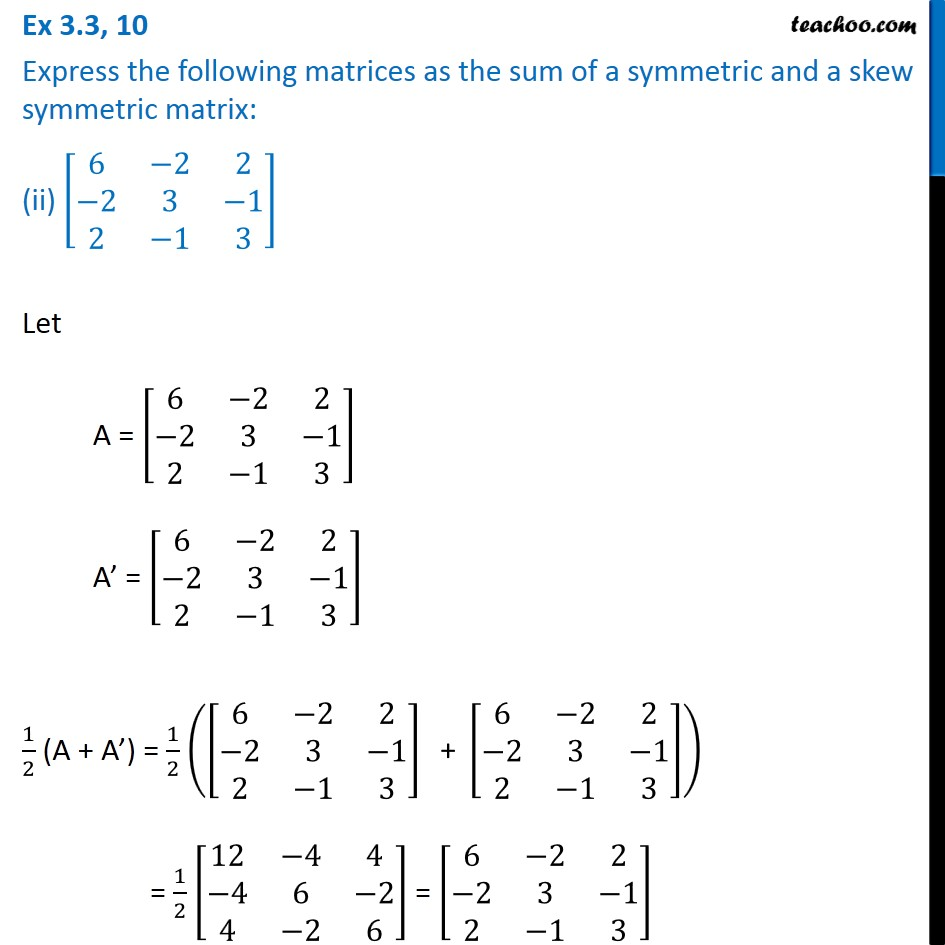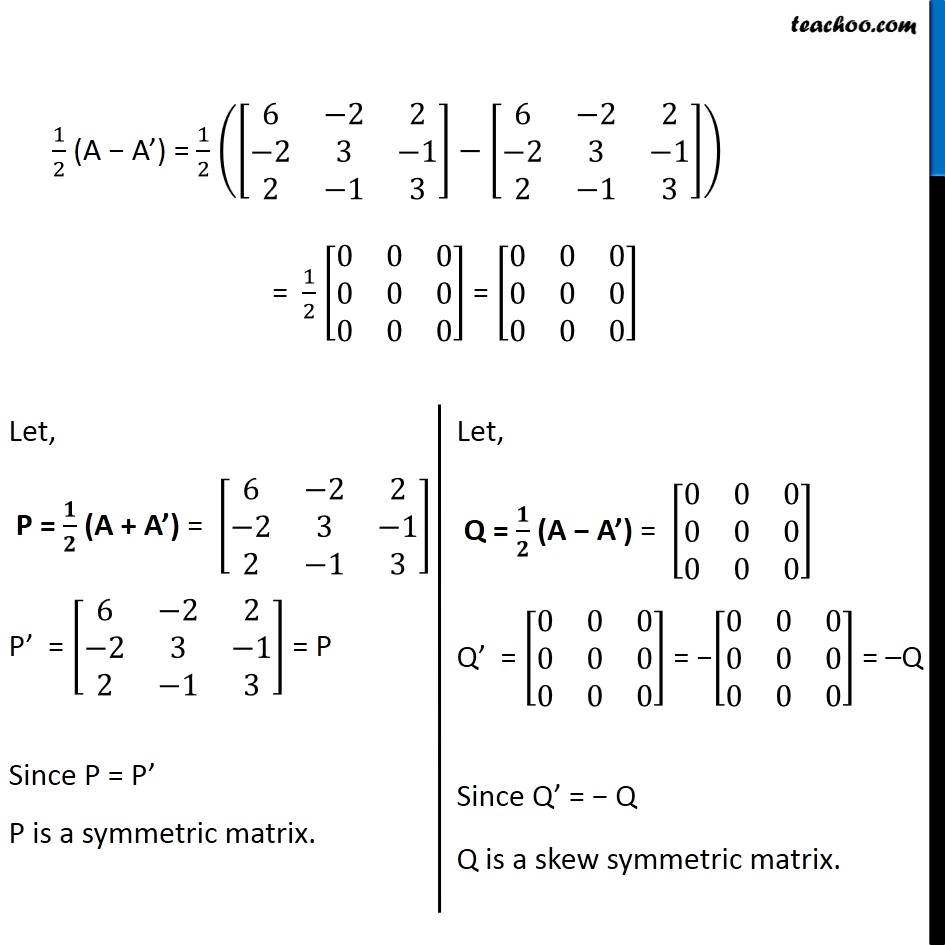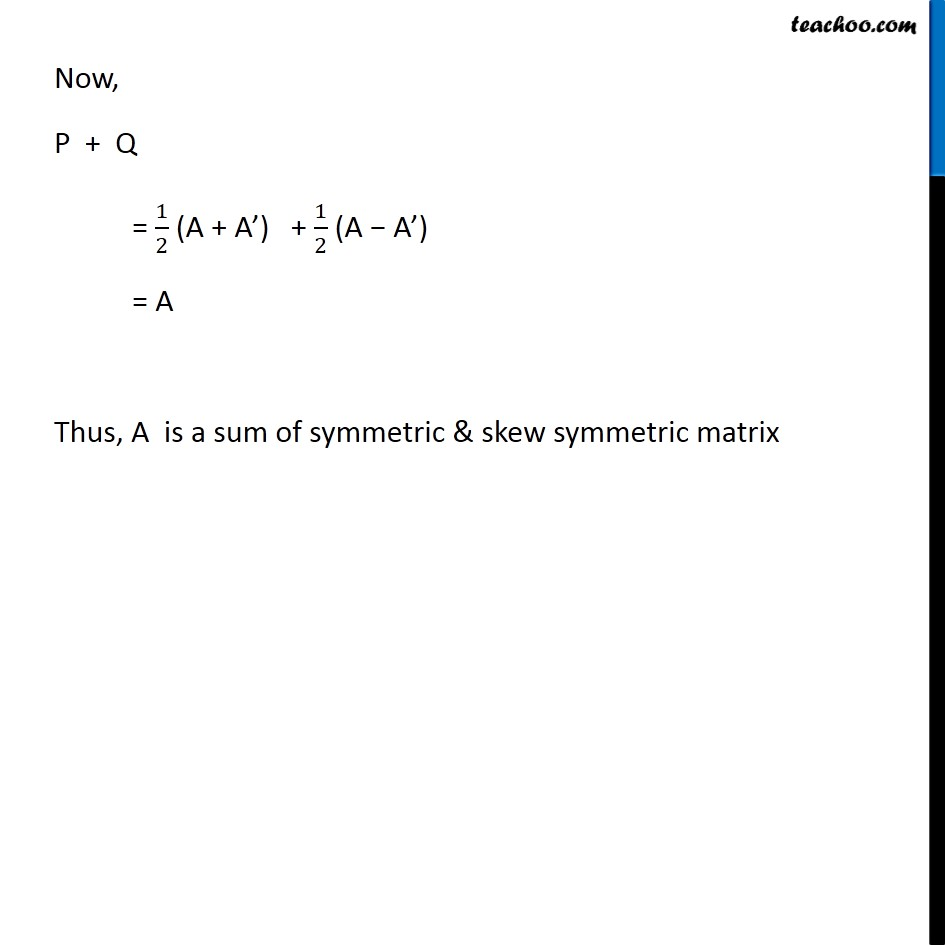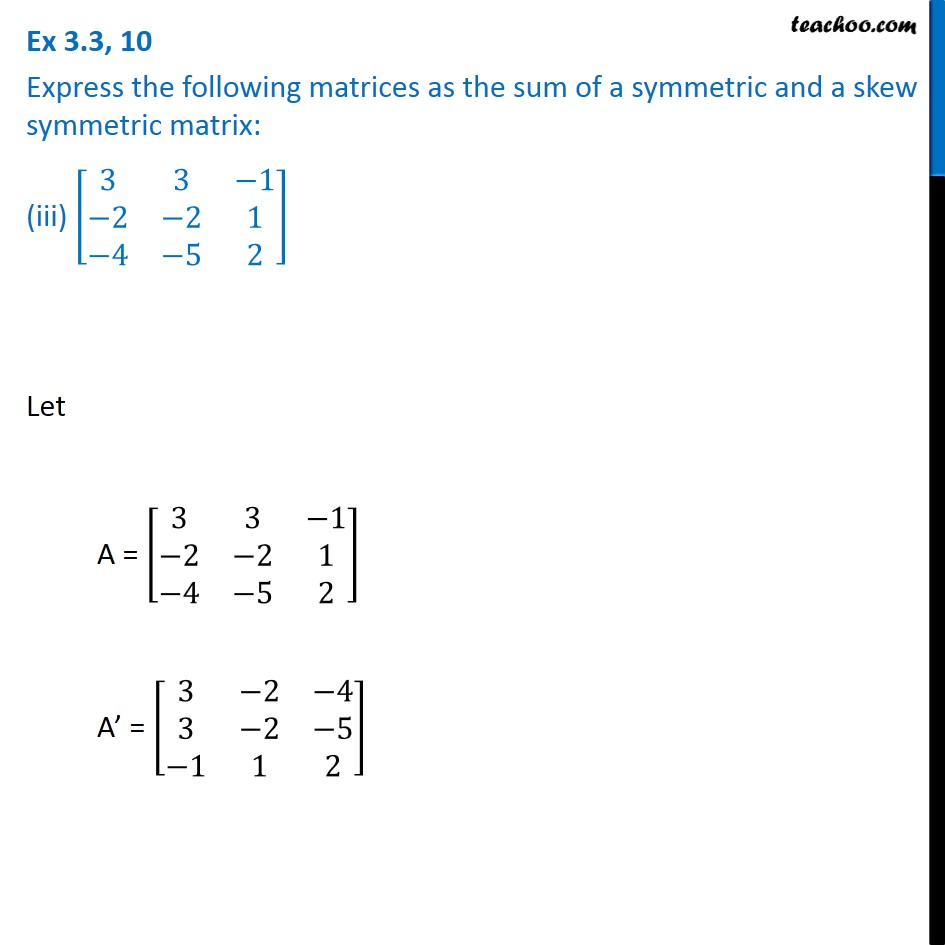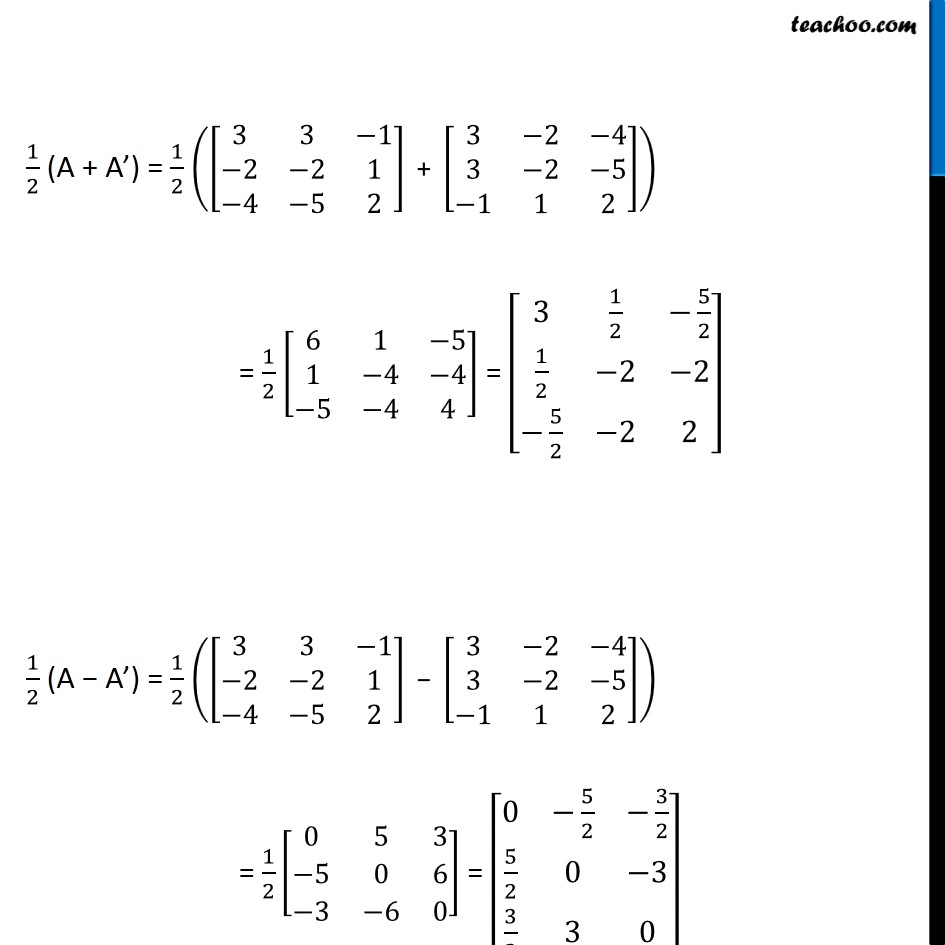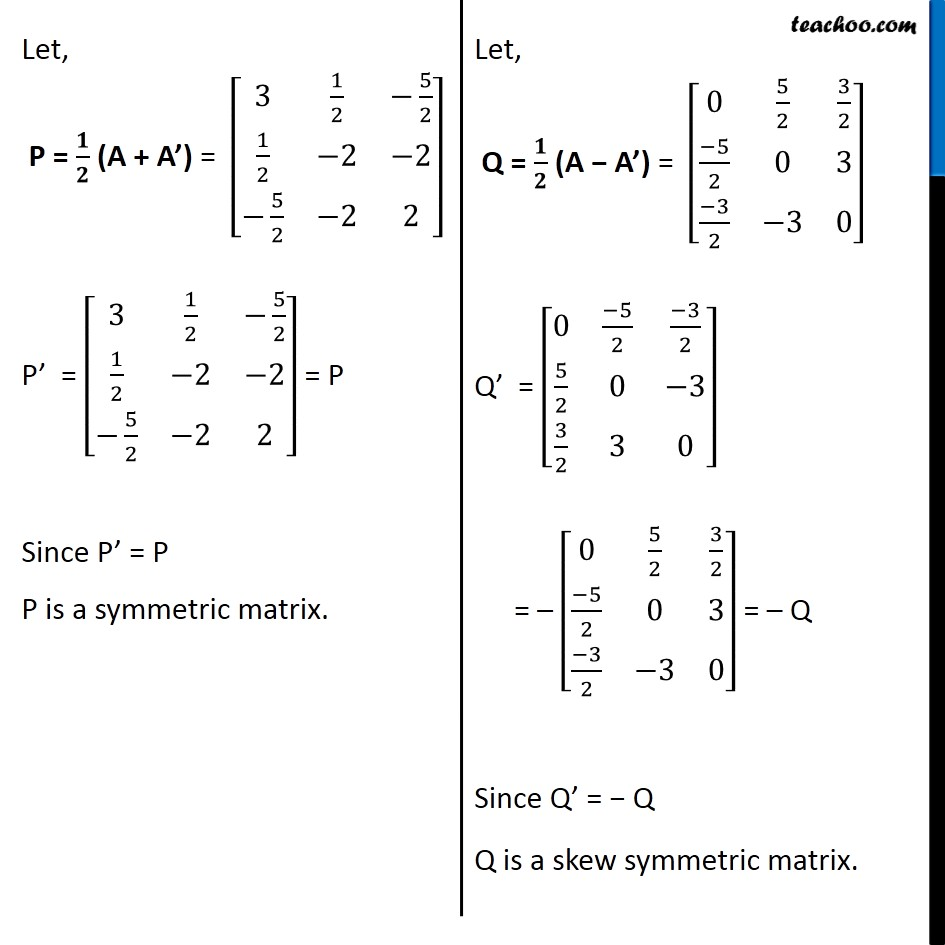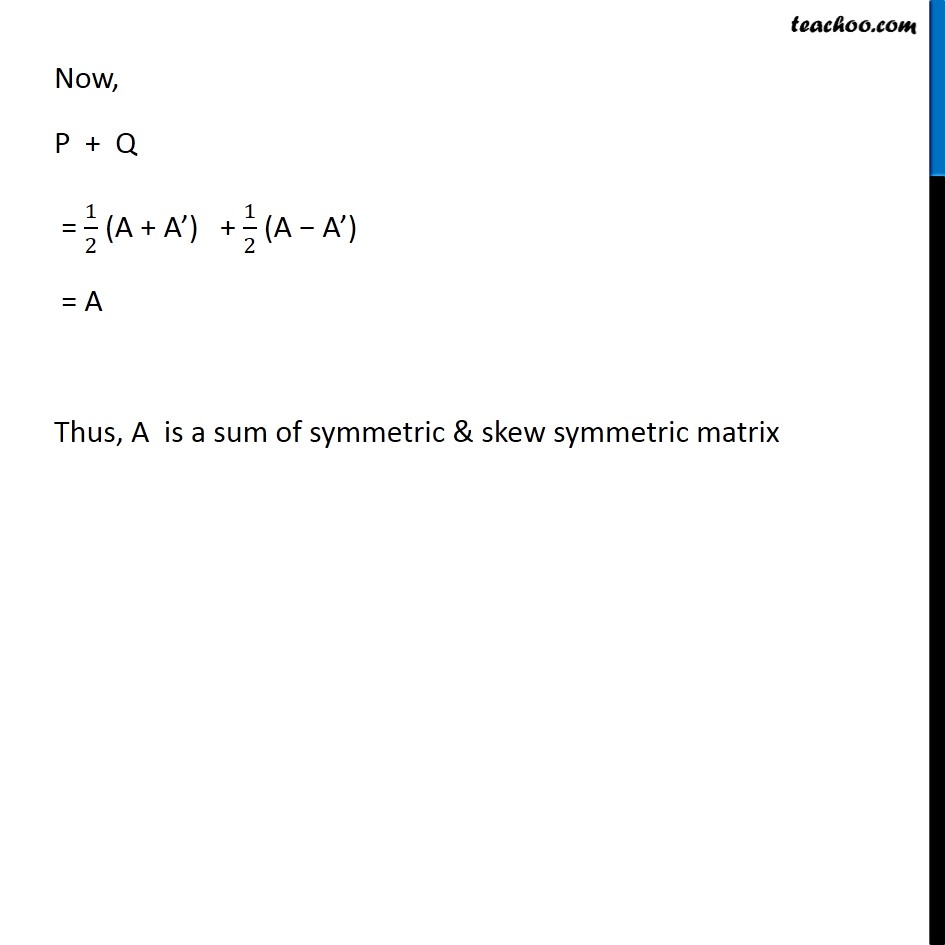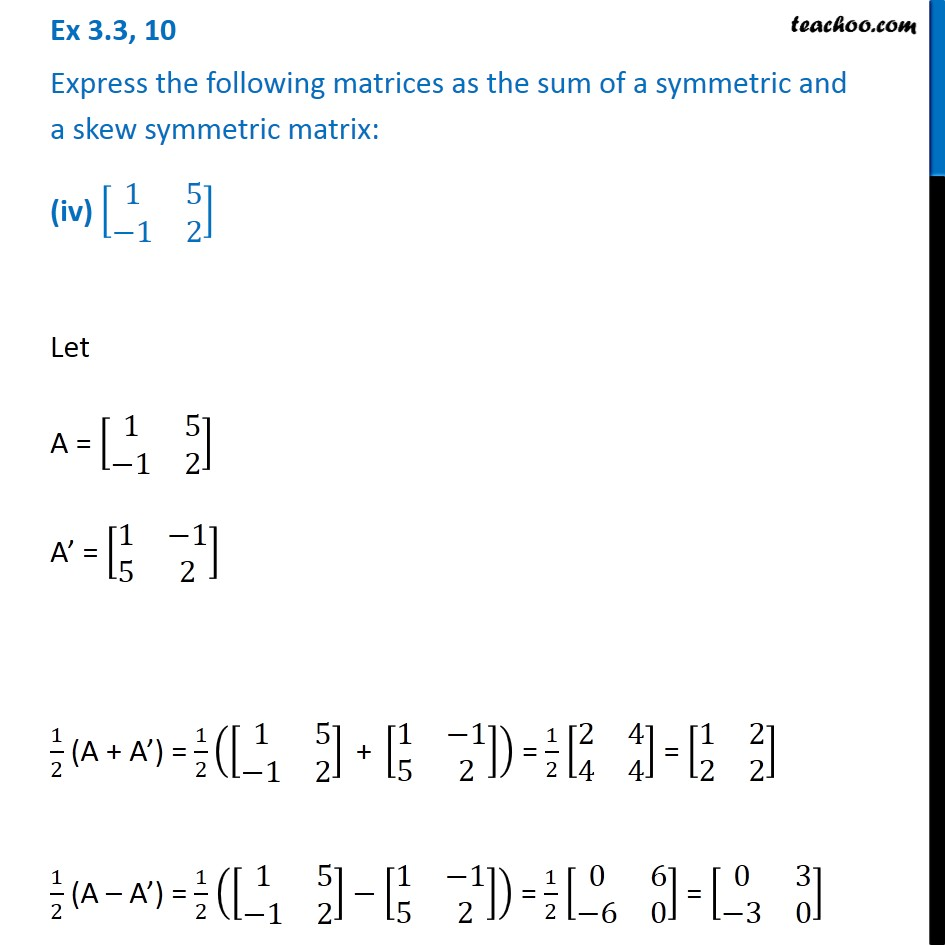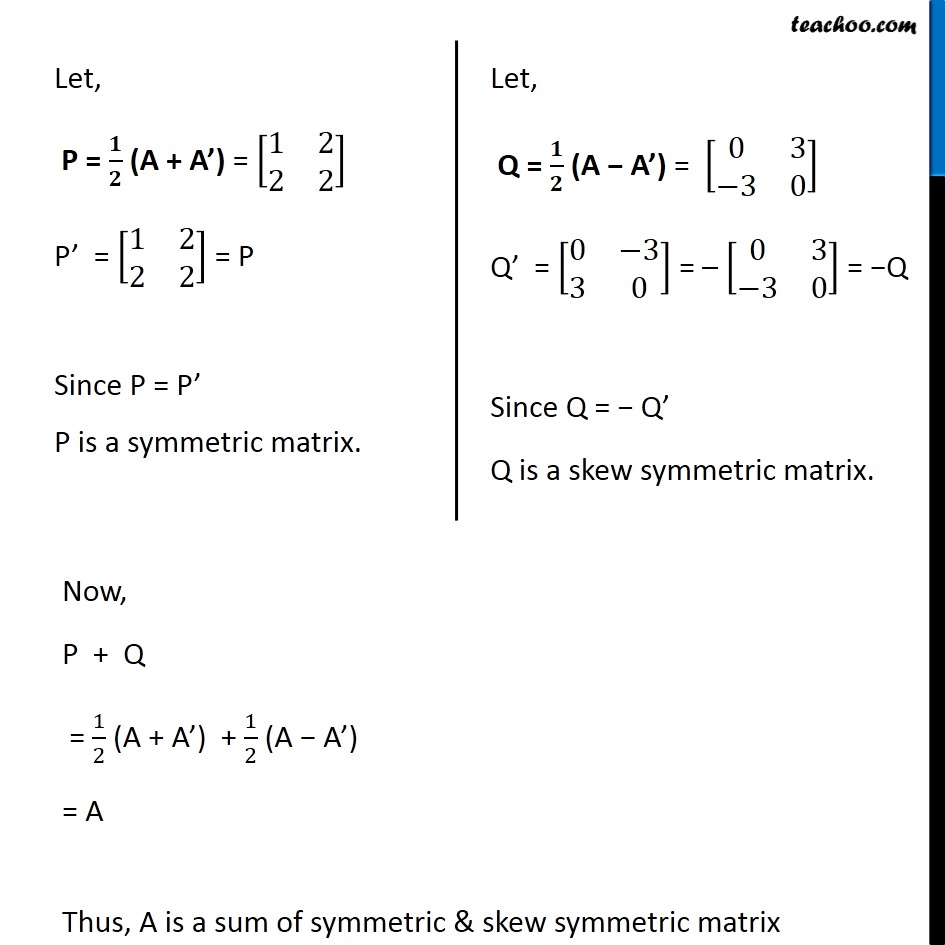1. Class 12
2. Important Questions for exams Class 12
3. Chapter 3 Class 12 Matrices

Transcript

Ex 3.3, 10 Express the following matrices as the sum of a symmetric and a skew symmetric matrix: (i) [■8(3&5@1&−1)] Let A = [■8(3&5@1&−1)] A’ = [■8(3&1@5&−1)] 1/2 (A + A’) = 1/2 ([■8(3&5@1&−1)]+ [■8(3&1@5&−1)]) = 1/2 [■8(6&6@6&−2)] = [■8(3&3@3&−1)] 1/2 (A – A’) = 1/2 ([■8(3&5@1&−1)]" − " [■8(3&1@5&−1)]) = 1/2 [■8(0&4@−4&0)] = [■8(0&2@−2&0)] Let, P = 𝟏/𝟐 (A + A’) = [■8(3&3@3&−1)] P’ = [■8(3&3@3&−1)] = P Since P‘ = P P is a symmetric matrix. Let, Q = 𝟏/𝟐 (A − A’) = [■8(0&2@−2&0)] Q’ = [■8(0&−2@2&0)] = – [■8(0&2@−2&0)]= −Q Since Q’ = − Q Q is a skew symmetric matrix. Now, P + Q = 1/2 (A + A’) + 1/2 (A − A’) = A Thus, A is a sum of symmetric & skew symmetric matrix Ex 3.3, 10 Express the following matrices as the sum of a symmetric and a skew symmetric matrix: (ii) [■8(6&−2&2@−2&3&−1@2&−1&3)] Let A = [■8(6&−2&2@−2&3&−1@2&−1&3)] A’ = [■8(6&−2&2@−2&3&−1@2&−1&3)] 1/2 (A + A’) = 1/2 ([■8(6&−2&2@−2&3&−1@2&−1&3)]" + " [■8(6&−2&2@−2&3&−1@2&−1&3)]) = 1/2 [■8(12&−4&4@−4&6&−2@4&−2&6)] = [■8(6&−2&2@−2&3&−1@2&−1&3)] 1/2 (A − A’) = 1/2 ([■8(6&−2&2@−2&3&−1@2&−1&3)]−[■8(6&−2&2@−2&3&−1@2&−1&3)]) = 1/2 [■8(0&0&0@0&0&0@0&0&0)] = [■8(0&0&0@0&0&0@0&0&0)] Let, P = 𝟏/𝟐 (A + A’) = [■8(6&−2&2@−2&3&−1@2&−1&3)] P’ = [■8(6&−2&2@−2&3&−1@2&−1&3)] = P Since P = P’ P is a symmetric matrix. Let, Q = 𝟏/𝟐 (A − A’) = [■8(0&0&0@0&0&0@0&0&0)] Q’ = [■8(0&0&0@0&0&0@0&0&0)] = −[■8(0&0&0@0&0&0@0&0&0)] = –Q Since Q’ = − Q Q is a skew symmetric matrix. Now, P + Q = 1/2 (A + A’) + 1/2 (A − A’) = A Thus, A is a sum of symmetric & skew symmetric matrix Ex 3.3, 10 Express the following matrices as the sum of a symmetric and a skew symmetric matrix: (iii) [■8(3&3&−1@−2&−2&1@−4&−5&2)] Let A = [■8(3&3&−1@−2&−2&1@−4&−5&2)] A’ = [■8(3&−2&−4@3&−2&−5@−1&1&2)] 1/2 (A + A’) = 1/2 ([■8(3&3&−1@−2&−2&1@−4&−5&2)]" + " [■8(3&−2&−4@3&−2&−5@−1&1&2)]) = 1/2 [■8(6&1&−5@1&−4&−4@−5&−4&4)] = [■8(3&1/2&−5/2@1/2&−2&−2@−5/2&−2&2)] 1/2 (A − A’) = 1/2 ([■8(3&3&−1@−2&−2&1@−4&−5&2)]" − " [■8(3&−2&−4@3&−2&−5@−1&1&2)]) = 1/2 [■8(0&5&3@−5&0&6@−3&−6&0)] = [■8(0&−5/2&−3/2@5/2&0&−3@3/2&3&0)] Let, P = 𝟏/𝟐 (A + A’) = [■8(3&1/2&−5/2@1/2&−2&−2@−5/2&−2&2)] P’ = [■8(3&1/2&−5/2@1/2&−2&−2@−5/2&−2&2)] = P Since P’ = P P is a symmetric matrix. Let, Q = 𝟏/𝟐 (A − A’) = [■8(0&5/2&3/2@(−5)/2&0&3@(−3)/2&−3&0)] Q’ = [■8(0&(−5)/2&(−3)/2@5/2&0&−3@3/2&3&0)] = – [■8(0&5/2&3/2@(−5)/2&0&3@(−3)/2&−3&0)] = – Q Since Q’ = − Q Q is a skew symmetric matrix. Let, Q = 𝟏/𝟐 (A − A’) = [■8(0&5/2&3/2@(−5)/2&0&3@(−3)/2&−3&0)] Q’ = [■8(0&(−5)/2&(−3)/2@5/2&0&−3@3/2&3&0)] = – [■8(0&5/2&3/2@(−5)/2&0&3@(−3)/2&−3&0)] = – Q Since Q’ = − Q Q is a skew symmetric matrix. Ex 3.3, 10 Express the following matrices as the sum of a symmetric and a skew symmetric matrix: (iv) [■8(1&5@−1&2)] Let A = [■8(1&5@−1&2)] A’ = [■8(1&−1@5&2)] 1/2 (A + A’) = 1/2 ([■8(1&5@−1&2)]" + " [■8(1&−1@5&2)]) = 1/2 [■8(2&4@4&4)] = [■8(1&2@2&2)] 1/2 (A – A’) = 1/2 ([■8(1&5@−1&2)]−[■8(1&−1@5&2)]) = 1/2 [■8(0&6@−6&0)] = [■8(0&3@−3&0)] Let, P = 𝟏/𝟐 (A + A’) = [■8(1&2@2&2)] P’ = [■8(1&2@2&2)] = P Since P = P’ P is a symmetric matrix. Let, Q = 𝟏/𝟐 (A − A’) = [■8(0&3@−3&0)] Q’ = [■8(0&−3@3&0)] = – [■8(0&3@−3&0)] = −Q Since Q = − Q’ Q is a skew symmetric matrix. Now, P + Q = 1/2 (A + A’) + 1/2 (A − A’) = A Thus, A is a sum of symmetric & skew symmetric matrix

Chapter 3 Class 12 Matrices

Class 12
Important Questions for exams Class 12